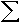## Step By Step Answers Example

1)

 20n=2 -3+3(n-1)

We could plug in all the numbers between 2 and 20 to get: 0+3+6+9+12+15+18+21+24+27+30+33+36+39+42+45+48+51+54
And then add them all together

OR
We plug in n=2 to get a2=0
We plug in n=20 to get a20=54
The number of terms, k = 20-2+1 so k=19
Then we use the formula k/2(a2+a20) to get: 513

2)
 8n=-1 7+3(n-1)

We could plug in all the numbers between -1 and 8 to get: 1+4+7+10+13+16+19+22+25+28
And then add them all together

OR
We plug in n=-1 to get a-1=1
We plug in n=8 to get a8=28
The number of terms, k = 8--1+1 so k=10
Then we use the formula k/2(a-1+a8) to get: 145

3)
 16n=3 -10+(n-1)

We could plug in all the numbers between 3 and 16 to get: -8+-7+-6+-5+-4+-3+-2+-1+0+1+2+3+4+5
And then add them all together

OR
We plug in n=3 to get a3=-8
We plug in n=16 to get a16=5
The number of terms, k = 16-3+1 so k=14
Then we use the formula k/2(a3+a16) to get: -21

4)
 13n=4 4+4/3(n-1)

We could plug in all the numbers between 4 and 13 to get: 8+28/3+32/3+12+40/3+44/3+16+52/3+56/3+20
And then add them all together

OR
We plug in n=4 to get a4=8
We plug in n=13 to get a13=20
The number of terms, k = 13-4+1 so k=10
Then we use the formula k/2(a4+a13) to get: 140